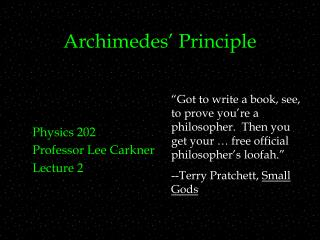DownloadDownload PresentationArchimedes’ Principle

# Archimedes’ Principle

Download Presentation## Archimedes’ Principle

- - - - - - - - - - - - - - - - - - - - - - - - - - - E N D - - - - - - - - - - - - - - - - - - - - - - - - - - -
##### Presentation Transcript

1. Archimedes’ Principle Physics 202 Professor Lee Carkner Lecture 2 “Got to write a book, see, to prove you’re a philosopher. Then you get your … free official philosopher’s loofah.” --Terry Pratchett, Small Gods

2. PAL #1 Fluids • Column of water to produce 1 atm of pressure • r = 1000 kg/m3 • h = P/rg = 10.3 m • Double diameter, pressure does not change • On Mars pressure would decrease

3. Archimedes’ Principle • The fluid exerts a force on the object • If you measure the buoyant force and the weight of the displaced fluid, you find: • An object in a fluid is supported by a buoyant force equal to the weight of fluid it displaces • Applies to objects both floating and submerged

4. Buoyancy

5. Will it Float? • What determines if a object will sink or float? • A floating object displaces fluid equal to its weight • A sinking object displaces fluid equal to its volume

6. Floating • How will an object float? • The denser the object, the lower it will float, or: • Example: ice floating in water, W=rVg Vi/Vw=rw/ri rw = 1024 kg/m3 and ri = 917 kg/m3

7. Iceberg

8. Ideal Fluids • Steady -- • Incompressible -- • Nonviscous -- • Irrotational -- • Real fluids are much more complicated • The ideal fluid approximation is usually not very good

9. Moving Fluids • Consider a pipe of cross sectional area A with a fluid moving through it with velocity v • Mass must be conserved so, • If the density is constant then, Av= constant = R = volume flow rate • Because the amount of fluid going in must equal the amount of fluid going out

10. Continuity • R=Av=constant is called the equation of continuity • You can use it to determine the flow rates of a system of pipes • Can’t lose or gain any material

11. Continuity

12. The Prancing Fluids • How can we keep track of it all? • The laws of physics must be obeyed • Neither energy nor matter can be created or destroyed

13. Bernoulli’s Equation • Consider a pipe that bends up and gets wider at the far end with fluid being forced through it • The work of the system due to lifting the fluid is, • The work of the system due to pressure is, Wp=Fd=pAd=DpDV=-(p2-p1)DV • The change in kinetic energy is, • Equating work and DKE yields, p1+(1/2)rv12+rgy1=p2+(1/2)rv22+rgy2

14. Fluid Flow

15. Consequences of Bernoulli’s Equation • Fast moving fluids exert less pressure than slow moving fluids • This is known as Bernoulli’s principle • Based on conservation of energy • Note that Bernoulli only holds for moving fluids

16. Constricted Flow

17. Bernoulli in Action • Blowing between two pieces of paper • Convertible top bulging out • Shower curtains getting sucked into the shower

18. Shower Physics

19. Lift • Consider a thin surface with air flowing above and below it • This force is called lift • If you can somehow get air to flow over an object to produce lift, what happens?

20. December 17, 1903

21. Deriving Lift • Consider a wing of area A, in air of density r • Use Bernoulli’s equation: • The difference in pressure is: pb-pt=1/2rvt2-1/2rvb2 • Pressure is F/A so: • L=Fb-Ft and so: • If the lift is greater than the weight of the plane, you fly

22. Summary: Fluid Basics • Density =r=m/V • Pressure=p=F/A • On Earth the atmosphere exerts a pressure and gravity causes columns of fluid to exert pressure • Pressure of column of fluid: p=p0+rgh • For fluid of uniform density, pressure only depends on height

23. Summary: Pascal and Archimedes • Pascal -- pressure on one part of fluid is transmitted to every other part • Hydraulic lever -- A small force applied for a large distance can be transformed into a large force over a short distance Fo=Fi(Ao/Ai) and do=di(Ai/Ao) • Archimedes -- An object is buoyed up by a force equal to the weight of the fluid it displaces • Must be less dense than fluid to float

24. Summary: Moving Fluids • Continuity -- the volume flow rate (R=Av) is a constant • fluid moving into a narrower pipe speeds up • Bernoulli p1+1/2rv12+rgy1=p2+1/2rv22+rgy2 • Slow moving fluids exert more pressure than fast moving fluids